# 1427 Mysterious Cat

This mysterious Halloween cat shares twelve clues that can help you solve its puzzle. Each clue is the products of two factors from 1 to 12 multiplied together. Will you be able to solve its mystery?Print the puzzles or type the solution in this excel file: 12 Factors 1419-1429

Now I’ll tell you a little bit about the puzzle number, 1427:

• 1427 is a prime number.
• Prime factorization: 1427 is prime.
• 1427 has no exponents greater than 1 in its prime factorization, so √1427 cannot be simplified.
• The exponent in the prime factorization is 1. Adding one to that exponent we get (1 + 1) = 2. Therefore 1427 has exactly 2 factors.
• The factors of 1427 are outlined with their factor pair partners in the graphic below.

How do we know that 1427 is a prime number? If 1427 were not a prime number, then it would be divisible by at least one prime number less than or equal to √1427. Since 1427 cannot be divided evenly by 2, 3, 5, 7, 11, 13, 17, 19, 23, 29, 31, or 37, we know that 1427 is a prime number.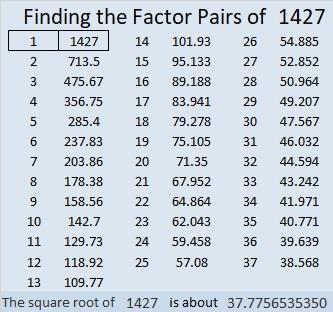1427 is 272 in BASE 25 because 2(25²) + 7(25) + 2(1) = 1427

# 1268 Halloween Cat Mystery

Cats can be quite mysterious. They are a favorite pet for many every day, even though suspicious stories abound about them on Halloween. Can you solve the mystery of this cat-like puzzle?Print the puzzles or type the solution in this excel file: 10-factors-1259-1270

Now I’ll share a few facts about the number 1268:

• 1268 is a composite number.
• Prime factorization: 1268 = 2 × 2 × 317, which can be written 1268 = 2² × 317
• The exponents in the prime factorization are 2 and 1. Adding one to each and multiplying we get (2 + 1)(1 + 1) = 3 × 2  = 6. Therefore 1268 has exactly 6 factors.
• Factors of 1268: 1, 2, 4, 317, 634, 1268
• Factor pairs: 1268 = 1 × 1268, 2 × 634, or 4 × 317
• Taking the factor pair with the largest square number factor, we get √1268 = (√4)(√317) = 2√317 ≈ 35.6089928² + 22² = 1268

1268 is the hypotenuse of a Pythagorean triple:
300-1232-1268 calculated from 28² – 22², 2(28)(22), 28² + 22².
It is also 4 times (75-308-317)

# 1052 A Mysterious Purple Cat for Josephine

My very brilliant friend, Josephine likes cats and her favorite color is purple. Hence, this is a purple cat puzzle made especially for her. It’s a mystery level puzzle so its difficulty level is a big secret. This puzzle only requires skills in multiplication and division. I’m sure it will be no match for Josephine, who can easily handle more advanced mathematics such as calculus. Josephine is also fluent in English, Chinese, Spanish, French, Arabic, and Tajiki.  She is very busy, so hopefully, she’ll be able to find the time to spend some time with this mysterious purple cat.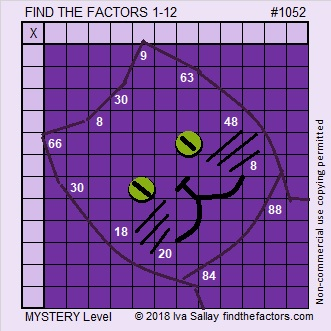Print the puzzles or type the solution in this excel file: 12 factors 1044-1053

If the colors in the puzzle distract you, here is the same puzzle in very plain black and white:Here is some information about the number 1052:

Its last two digits are 52, so it is divisible by 4.

• 1052 is a composite number.
• Prime factorization: 1052 = 2 × 2 × 263, which can be written 1052 = 2² × 263
• The exponents in the prime factorization are 2 and 1. Adding one to each and multiplying we get (2 + 1)(1 + 1) = 3 × 2  = 6. Therefore 1052 has exactly 6 factors.
• Factors of 1052: 1, 2, 4, 263, 526, 1052
• Factor pairs: 1052 = 1 × 1052, 2 × 526, or 4 × 263
• Taking the factor pair with the largest square number factor, we get √1052 = (√4)(√263) = 2√263 ≈ 32.434549480453709548485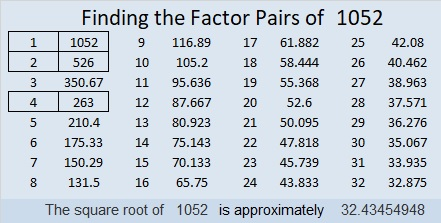1052 is palindrome 282 in BASE 21 because 2(21²) + 8(21) + 2(1) = 1052

# 928 Halloween Cat

This cat has arrived just in time for Halloween. Find the factors that go with the clues in the grid to make this Halloween Cat puzzle a multiplication table:Print the puzzles or type the solution on this excel file: 12 factors 923-931

Now let me tell you a little about the number 928.

It is the sum of four consecutive prime numbers:
227 + 229 + 233 + 239 = 928
and the sum of eight other consecutive prime numbers:
101 + 103 + 107 + 109 + 113 + 127 + 131 + 137 = 928

It is the sum of two squares:
28² + 12² = 928
That means 928 is the hypotenuse of a Pythagorean triple:
640-672-928 which is 28² – 12², 2(28)(12), 28² + 12²

Here’s how 928 looks in a few other bases:
It’s 565 in BASE 13, because 5(169) + 6(13) + 5(1) = 928.
It’s 4A4 in BASE 14 (A is 10 base 10), because 4(196) + 10(14) + 4(1) = 928.
It’s TT in BASE 31 (T is 29 base 10), because 29(31) + 29(1) = 29(32) = 928.
It’s T0 in BASE 32, because 29(32) = 928.

• 928 is a composite number.
• Prime factorization: 928 = 2 × 2 × 2 × 2 × 2 × 29, which can be written 732 = 2⁵ × 29
• The exponents in the prime factorization are 5 and 1. Adding one to each and multiplying we get (5 + 1)(1 + 1) = 6 × 2 = 12. Therefore 928 has exactly 12 factors.
• Factors of 928: 1, 2, 4, 8, 16, 29, 32, 58, 116, 232, 464, 928
• Factor pairs: 928 = 1 × 928, 2 × 464, 4 × 232, 8 × 116, 16 × 58, or 29 × 32
• Taking the factor pair with the largest square number factor, we get √928 = (√16)(√58) = 4√58 ≈ 30.463092423.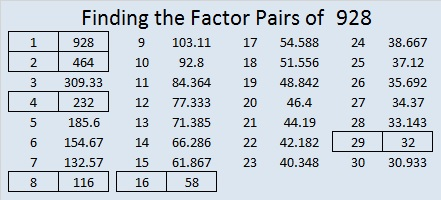# 664 The Back of a Halloween Cat’s Head

664  in base 12 is the palindrome 474. Note that 4(144) + 7(12) + 4(1)= 664.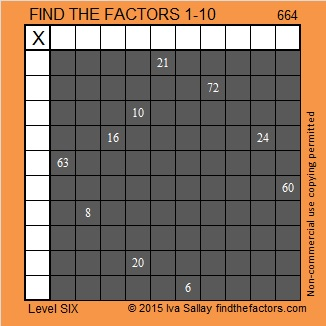Print the puzzles or type the solution on this excel file: 10 Factors 2015-10-26

—————————————————————————————————

• 664 is a composite number.
• Prime factorization: 664 = 2 x 2 x 2 x 83, which can be written 664 = (2^3) x 83
• The exponents in the prime factorization are 3 and 1. Adding one to each and multiplying we get (3 + 1)(1 + 1) = 4 x 2 = 8. Therefore 664 has exactly 8 factors.
• Factors of 664: 1, 2, 4, 8, 83, 166, 332, 664
• Factor pairs: 664 = 1 x 664, 2 x 332, 4 x 166, or 8 x 83
• Taking the factor pair with the largest square number factor, we get √664 = (√4)(√166) = 2√166 ≈ 25.768197.—————————————————————————————————Ricardo tweeted the solution: